# Marginal Profit

An additional cost or profit earned when one additional, or marginal, unit is produced and sold

When one additional, or marginal, unit is produced and sold, a firm or individual earns a marginal profit, also known as MP. The additional cost or profit earned by generating the following unit is referred to as "marginal."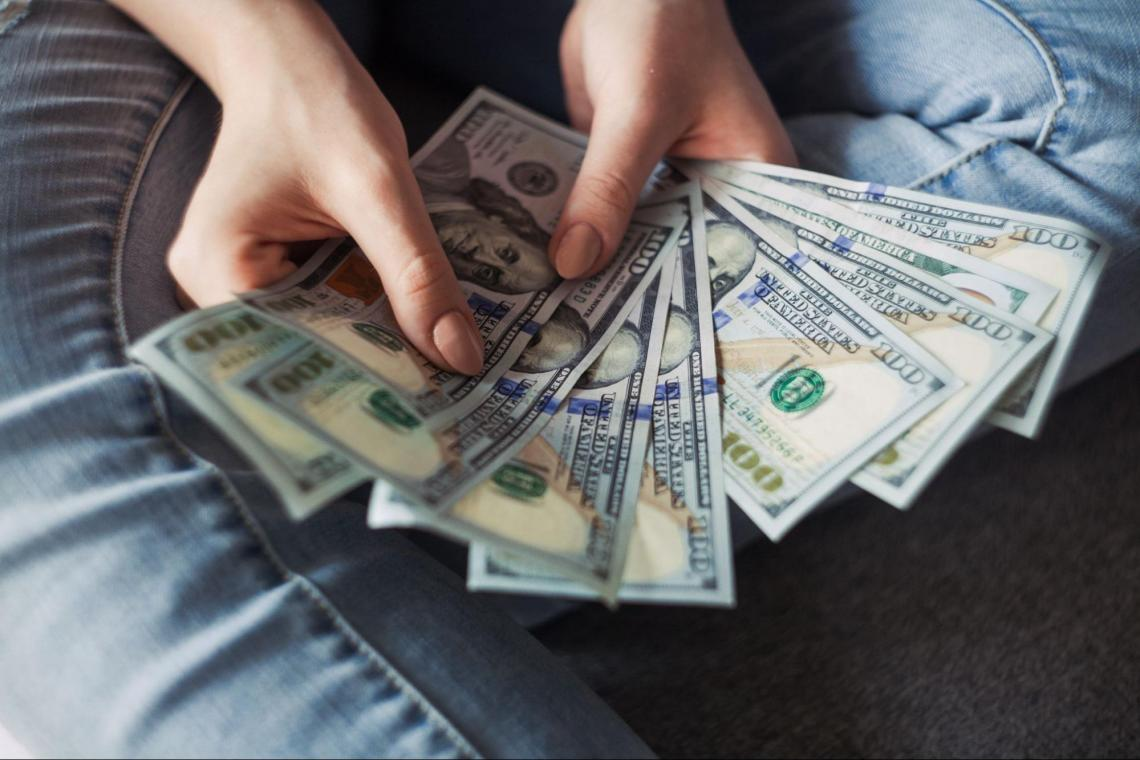The additional money earned is known as marginal revenue, whereas the higher expense of producing one additional unit is known as marginal cost

The difference between marginal cost and marginal revenue is called marginal profit.

Managers benefit from analyzing it because it helps them decide whether to grow production, slow down or cease output entirely if the firm reaches its shutdown point.

According to the profit maximization rule, a company's overall profitability will be maximized when marginal cost equals marginal revenue or when marginal profit is exactly zero.

Marginal revenue refers to the additional money generated when an extra unit is produced and sold. Marginal cost is the change in production cost when the quantity produced increases by one unit.

Based on predicted revenue and expenditures, businesses use the MP to decide whether to expand, downsize, or stop producing a good. A company's marginal profit is the profit gained when it sells one additional unit of production.

It is measured as marginal revenue minus marginal cost (i.e., the amount of revenue a corporation earns from selling one additional production item) (i.e., the cost of producing one more unit of production).

Firms must understand MP to determine how much they should produce, especially in a competitive market.

Understanding the basics

It differs from average profit, net profit, and other profitability measurements in that it considers the profit that can be made by producing one more unit.It considers the amount of production since when a company grows, its cost structure changes, and its profitability can increase or decrease as production ramps up, depending on economies of scale.

The term "economies of scale" refers to a situation where MP rises as production scale rises. Economies of scale result when average costs decrease as the scale of production increases.

As the scale expands to the point where average costs stagnate and then begin to rise again, it will eventually become a diseconomy of scale.

At this point, the company is experiencing scaling inefficiencies. As a result, businesses will tend to increase production only until marginal cost equals marginal revenue or until it equals zero.

In other words, when marginal cost and marginal revenue are both zero, creating an additional unit yields no further profit.

If a firm's MP becomes negative, its management may opt to reduce production, temporarily halt production, or exit the business altogether if positive marginal earnings do not appear to be returning.

Operating profit, gross profit, marginal profit, and net profit are ways businesses measure the money they make. However, these profits are computed differently and provide information about a company's operations and future.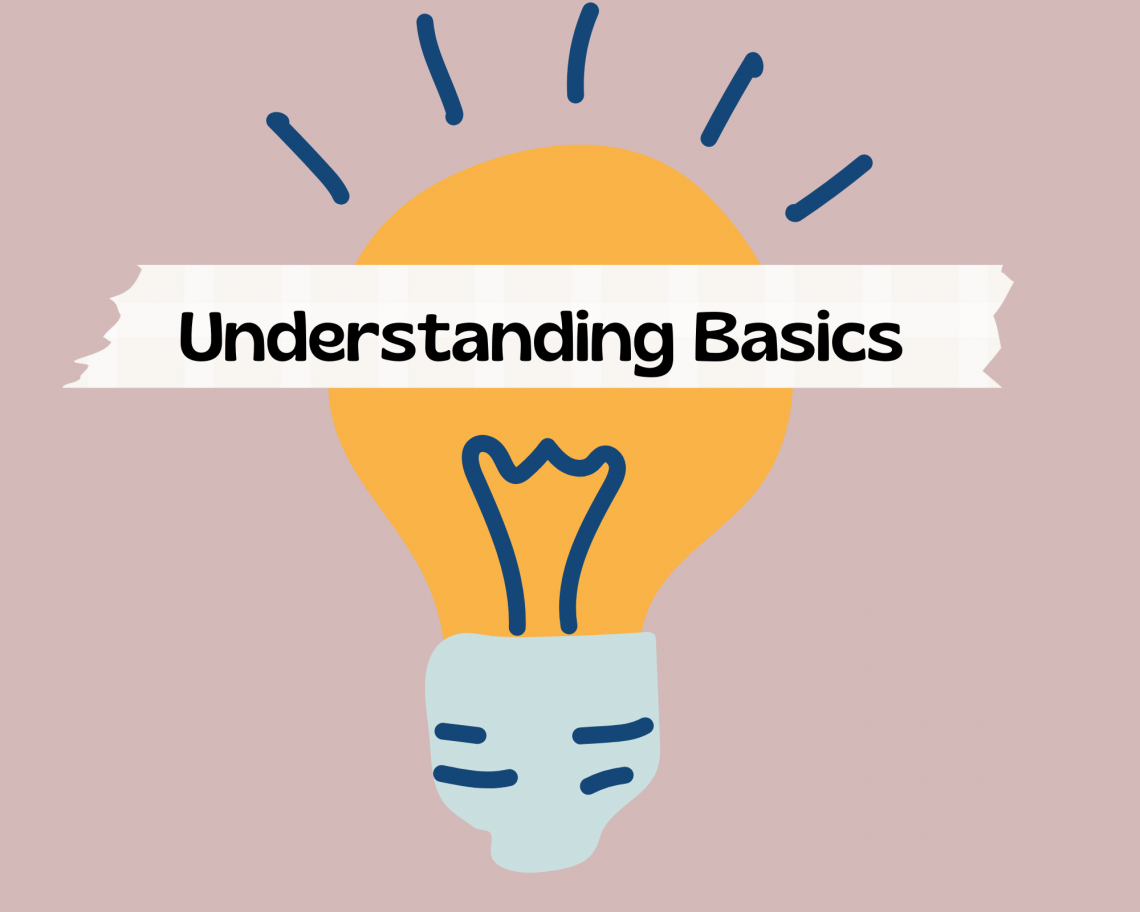Instead of focusing on the company's cost structure, the measure we're discussing concerns the profit created by an extra output unit.

Because a company's profitability fluctuates as the quantity of output changes, this measure accounts for the scale of production.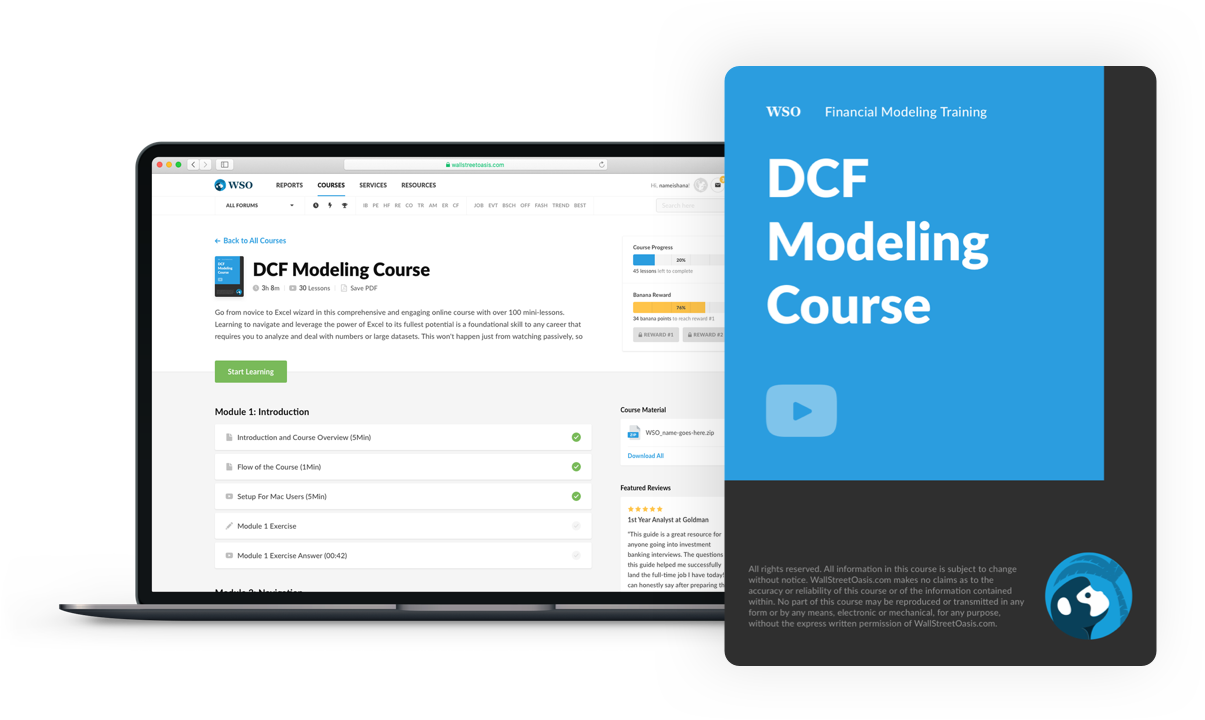### Everything You Need To Master DCF Modeling

To Help You Thrive in the Most Prestigious Jobs on Wall Street.

## Relevant Definitions and Formulas

These are the definitions and formulas that are key to understanding this concept. It's crucial to distinguish between the various "profits," as mixing them up can lead to bad decision-making.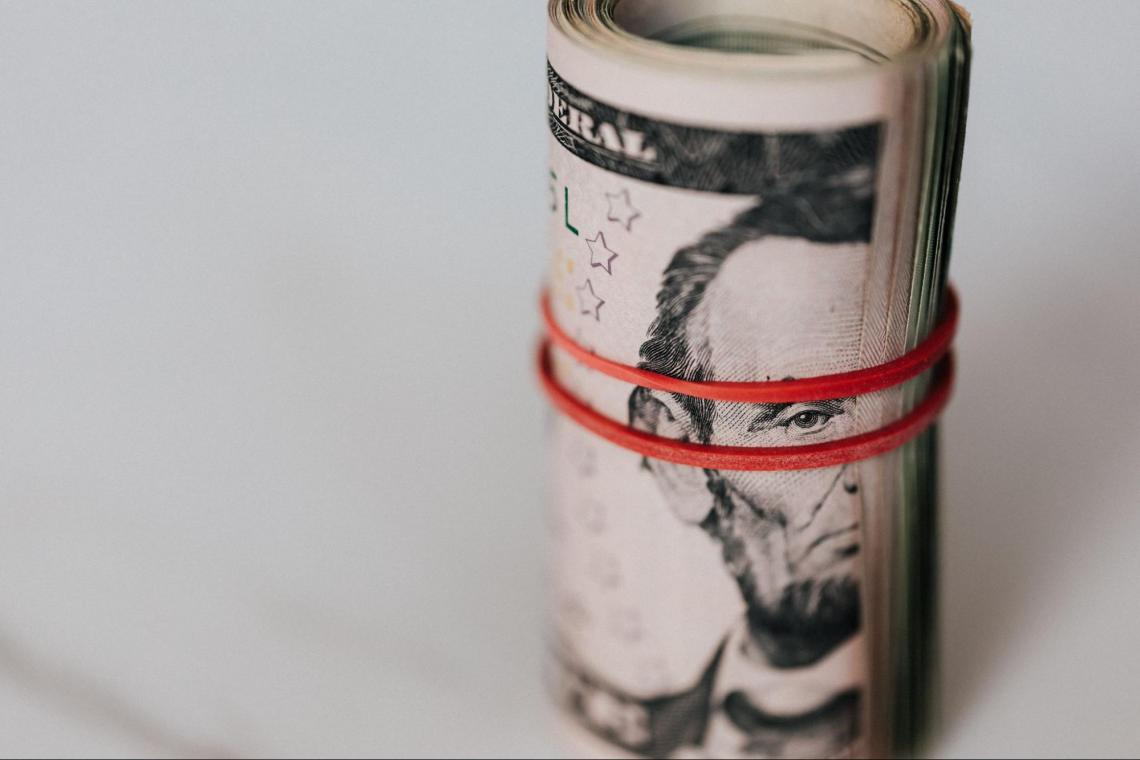Let's have a look at some of them:

• The profit made by producing and selling one extra unit of production is known as MP.

• The average profit per unit of production is known as the average profit.

• Total profit: total profit is the total amount earned from selling all goods produced.

Let's establish the linked income and cost measurements before writing down some equations to find the marginal profit, average profit, and total profit.

a) Definitions of revenue

• The amount received from selling one extra manufacturing unit is known as marginal revenue.

• Average revenue is the amount received on average for each unit sold.

b) Definitions of costs

• The amount spent to manufacture one more unit of output is known as marginal cost.

• The average cost per unit of output is known as the average cost.

• Total cost: the total amount spent on everything generated is referred to as total cost.

The marginal revenue formula is:

Marginal Revenue (MR) = Change in Total Revenue/Change in Total Quantity

The marginal cost formula is:

Marginal Cost (MC) = (Change in Costs)/(Change in Quantity)

The formula for profit on an additional unit is:

Marginal Profit (MP) = Marginal Revenue (MR) – Marginal Cost (MC)

The total profit formula is:

Total Profit (TP) = Total Revenue (TR) – Total Cost (TC)

The average profit formula is:

Average Profit = (Total Revenue – Total Cost)/Total Quantity

The formula for total revenue:

Total Revenue = Number of units sold x Selling price per unit

The formula for total cost:

Total Cost = Total fixed cost + Total variable cost

## How Do I Calculate MP?

The expense of producing one additional unit is called marginal cost (MC), and the money generated for producing one additional unit is called marginal revenue (MR).MP is expressed as the difference between the marginal revenue and the marginal cost as it relates to a sale of a unit. The formula is expressed as follows:

Marginal profit (MP) = Marginal revenue (MR) - marginal cost (MC)

In modern microeconomics, competing companies prefer to create units until marginal cost equals marginal revenue (MC=MR), leaving the producer with a zero MP.

There is no opportunity for economic profit in perfect competition because competition will always force the selling price down to marginal cost, and a firm will function until marginal revenue equals marginal cost.

Thus, not only does MC = MP = price, but also MC = MR = price.

If a company can't compete on price and has to operate at a loss (negative MP), it will eventually shut down. A firm's profit maximization happens when it produces up to the point when marginal cost equals marginal revenue and MP equals zero.

To maximize profit, businesses manufacture goods until marginal cost equals marginal revenue. As a result, the producer effectively has no MP.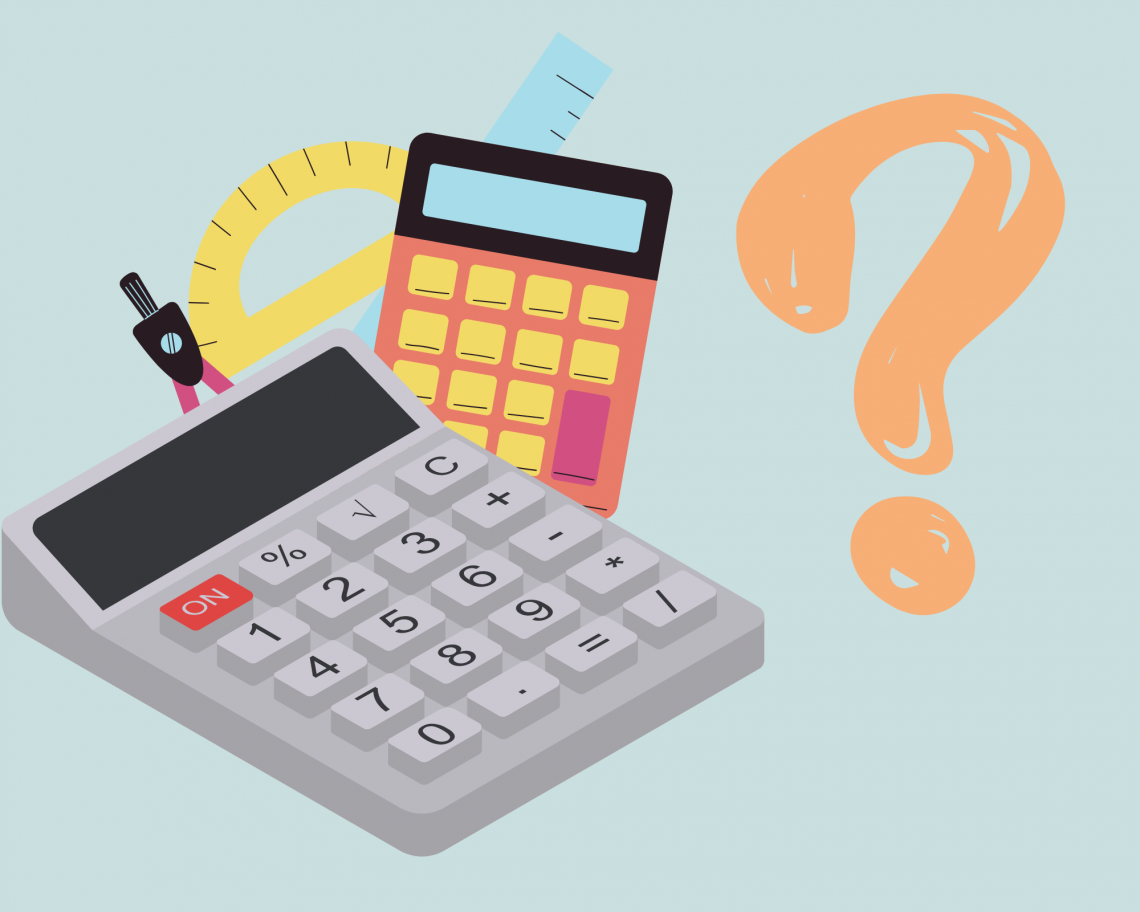### Special Considerations

It's vital to remember that marginal profit simply accounts for the profit made by producing one more item, not a company's overall profitability.

To put it another way, a company should stop producing when creating one more unit starts to decrease total profitability.Marginal cost is affected by several factors, including:

• Labor (and labor circumstances, such as unionization or shortages)

• Supplies or raw materials which are prone to inflation

• Interest on a loan

• Taxes

Fixed expenditures, also known as sunk costs, should not be included in the computation of MP because they do not change with the number of units produced.

Sunk costs are expenses that cannot be recovered, such as the cost of constructing a manufacturing plant or purchasing a piece of equipment.

Sunk costs are excluded from its analysis since it only considers the benefit from one more unit produced, not the money spent on non-recoverable costs like plant and equipment.

However, the psychological predisposition to incorporate fixed costs is difficult to overcome, and analysts often fall prey to the sunk cost fallacy, resulting in poor and frequently expensive management decisions.In actuality, many businesses optimize marginal earnings to the point where they always equal zero. Because of technical frictions, regulatory and legal contexts, and knowledge lags and asymmetries, very few markets approach ideal competition.

Managers of a company may not be aware of their marginal costs and revenues in real-time, which means they must frequently make production decisions in hindsight and forecast the future.

To be able to ramp up production without interruption when demand increases, many businesses run below their maximum capacity utilization.

A company should create as many units as possible to maximize revenues, but production expenses are likely to rise when production ramps up.The production level is optimal when MP is zero (i.e., when the marginal cost of producing one additional unit equals the marginal revenue it will generate). Production should be reduced if costs cause the MP to fall below zero.

Significance

Fixed costs and other variable costs not directly related to manufacturing are excluded.

If a corporation decides to maintain an attorney on retainer for $30,000 per year, adding another unit of production will not affect the retainer. The attorney's expense will not be factored into the MP in this scenario. Consider the case where an employee only makes 900 ice pops daily, although each employee can make 1,200. We exclude the employee's cost from the MP calculation until we reach the projected production capacity of 1,200 popsicles and surpass that production quantity by one extra item. Producing items becomes more expensive as a company approaches the upper limit of its available production capacity due to increased maintenance and overtime costs, which reduces the number of incremental sales that can be achieved. As a result, businesses will only expand output until MP equals zero. Producing an extra unit is not economically viable since the corporation will gain no more profit if the MP is zero.When Should a Business Close If It Is Making a Marginal Profit? Generally, a business should stay open if it's above breakeven. However, if the firm's MP is negative at all production levels, the wisest course of action is to stop all production for the time being rather than continue to produce units at a loss. What Are Economies of Scale? Economies of scale are situations where increasing production lowers the average cost per unit. In such instances, when more units are produced, the MP will rise until average costs become constant. What is the Maximum Profit Margin? The method for calculating maximum profit margin represents a step-change view of a company's performance. It may not apply to businesses where the cost of items sold is the most significant cost incurred during production.Typically, this method considers all revenue and expenses. A company's MP rate will collapse in the event of a considerable rise in costs to boost production. Consider a solar panel manufacturer that manufactures 200 solar panels for$100 each. The materials in each unit cost $40, and the MP on the 200th unit is$60.

The MP for the 201st item is -$29,940 if the corporation plans to buy a minor competitor for$30,000.

### Methods to Boost Your Profit Margin

Profitability can be improved by increasing revenue, lowering costs and expenses, or a combination of the two. Here are a few pointers to help you achieve your optimal profit margin.• Reduce operating costs, including office space and utilities, materials, supplies, salary and benefits, employee expenditures, insurance, equipment repair, shipping, and company software.

Negotiate lower pricing, downgrade, initiate a hybrid working model, or delete any services that aren't necessary.

• Cut low-margin products or services and replace them with higher-margin ones: You can do a break-even analysis to see if a product is genuinely lucrative.
You can get ideas from other companies or research high-margin items in your field. In any event, you'll have to weigh your intended selling price against your cost of goods sold and operating expenses.

• Experiment with multiple product pricing approaches, such as value-based pricing or cost-plus pricing, to fine-tune your price strategy. The impact of product pricing on demand may surprise you.

• Build brand loyalty: Engaging with customers regularly and expressing gratitude has a measurable impact on sales and customer retention. You can lower your advertising expenditures by retaining more clients.

## What is the Total Profit?

Let's start by adding up the revenue. We sold 50 shirts for $30 each, for a total of$1500. Then we sold 30 more for $20 apiece, for a total of$600. Finally, we sold 50 for $15, for a total of$750. As a result, the total revenue is $2850 ($1500 + $600 +$750).

Let's now add up all of our expenses. There are two types of expenses in this situation. The first is a fixed cost, which does not change depending on the quantity produced.

The machine costs $200. The variable expenses, or those that vary depending on the volume produced, are$2 per shirt or $2 x 130 =$260 overall. As a result, our total costs are $200 +$260 = $460.Finally, the overall profit is$2850 – $460 =$2390, which is revenue minus expenses.

(1) What is the average profit on each T-shirt?

The overall profit is $2390, and the number of units produced is 130. As a result, the average profit is$2390 divided by 130, or $18.38. (2) What is the MP on the first, twentieth, fifty-first, and the hundredth shirt sold? In tabular format, this will be easier to see: Example ShirtMarginal RevenueMarginal CostMarginal Profit 130202-172 2030228 5030228 6020218 10015213 The first 50 shirts generate a marginal revenue of$30, the following 30 shirts generate a marginal revenue of $20, and the remaining 50 shirts generate a marginal revenue of$15. The sale price is the only source of marginal revenue.

The first shirt necessitates equipment purchase, resulting in a relatively high marginal cost of $202 (the$200 machine + $2 for supplies and labor). The following 129 items all have a$2 marginal cost (the material and labor cost).

When you add them all up, you get a negative MP of –$172 (also known as a marginal loss), then shirts 2 to 50 have the same$28 MP, 51 to 80 have a MP of $18, and 81 to 130 have a MP of$13.

## In a Competitive Market, How is Profit Maximized when Marginal Profit is Zero?

It seems counterintuitive that profit is maximized when it is zero. It's important to note that total profit is not the same as MP and that average profit is not the same as MP either.

The difference between marginal cost and marginal revenue at each point, as well as the marginal cost and marginal revenue curves, is known as MP: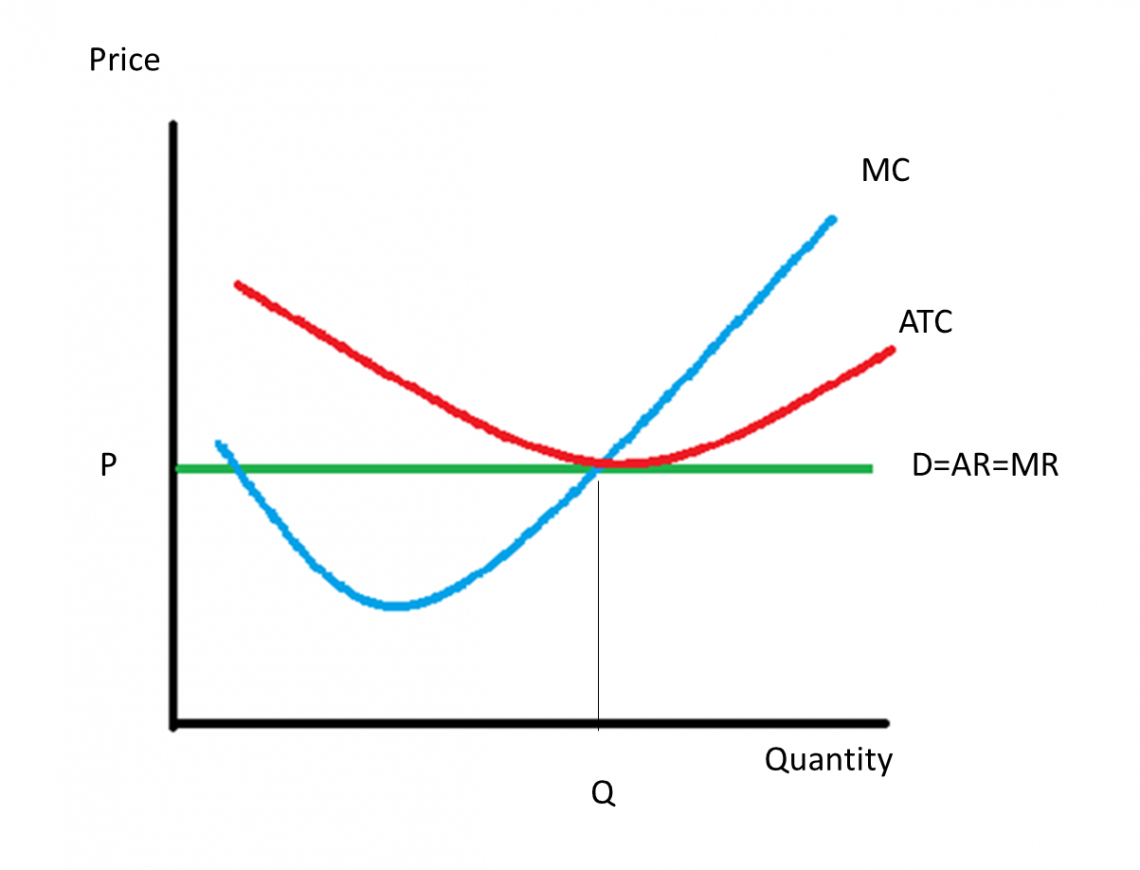(MC stands for marginal cost, ATC stands for the average total cost, D stands for demand, AR stands for average revenue, MR stands for marginal revenue, P stands for market price, and Q stands for quantity produced.)

The green line (marginal revenue, i.e., the selling price) is above the blue line (marginal cost) in this diagram, indicating profit.

If the blue line were extended to the price axis, there would be a marginal loss (the blue line would be above the green line) for the first little amount since the fixed costs are too high at such a low volume to generate a profit, just as we saw in our previous example.

The overall earnings are calculated by taking all of the profit in the area between the green and blue curves and subtracting the triangular area to the left where the blue curve is above the green curve.

This can be represented by a rectangle drawn by averaging the costs:(MC stands for marginal cost, ATC stands for an average total cost, D stands for demand, AR stands for average revenue, MR stands for marginal revenue, P stands for market price, C stands for cost, and Q stands for quantity produced.)

The average profit is represented by P – C (price minus average cost over that quantity), whereas the entire rectangle represents the overall earnings.

So, if the MP is zero, why is overall profit maximized? When the rectangle is as long as it can be.

Even if the final item sold creates no MP, each preceding item does, which means that selling until the marginal cost equals the marginal income leaves no money on the table-you've sold to every consumer from whom you can profit.

### Worked Example

A smartphone company knows that C(x)=6x^2+34x+2,500 is the cost of making x phones and that P = 60x is the demand function for their phones. Determine the marginal cost, marginal income, and MP of manufacturing 75 phones.To calculate marginal cost at 75 units, we take the cost function's derivative and then evaluate it at x=75.

Note that these marginal functions modify the slope of the original functions or the change per unit because they are derivative functions.

C'(x)=12x+34

C'(x)=12(75)+34

C'(x)=93

At x = 75, the marginal cost is $934, indicating that the additional cost of creating the 76th unit is$934.

We need to find a revenue function, take its derivative, and then evaluate the derivative at x = 75 to get marginal revenue at 75 units.

R(x)=Px, where p is the demand function and p = 60x is the revenue equation.

R(x)=x(60x)

R(x)=60x^2

We get R'(x)=120x by taking the revenue derivative to get marginal revenue.

Then, at x=75, we assess.

R'(x)=120(75)

R'(x)=9,000

At x=75, the marginal revenue is $9,000, implying that the additional revenue from selling the 76th unit is$9,000.

Finally, we must find a profit function, take its derivative, and evaluate the derivative at x=75 to solve for MP.

P(x)=R(x) - C(x) is the profit equation (x),

R stands for the revenue function we discovered before, and C stands for the cost function we were given.

P(x)=(60x^2​​)−(6x^2​​+34x+2,500)

P(x)=54x^2-34x-2,500

We get P'(x)=108x - 34 by taking the derivative of profit to derive MP.

Then, at x = 75, we find:

P'(x)=108(75) - 34

P'(x)= $8,066 At x=75, the MP is$8,066, implying that the additional profit associated with producing and selling the 76th unit is \$8,066.

Remember that marginal cost, revenue, and profit are all estimates. As a result, P'(x) will not always be the same as R'(x) - C'(x)### Everything You Need To Master Excel Modeling

To Help You Thrive in the Most Prestigious Jobs on Wall Street.

Researched and authored by Deeksha Pachauri LinkedIn

Reviewed and Edited by Sara De Meyer | LinkedIn